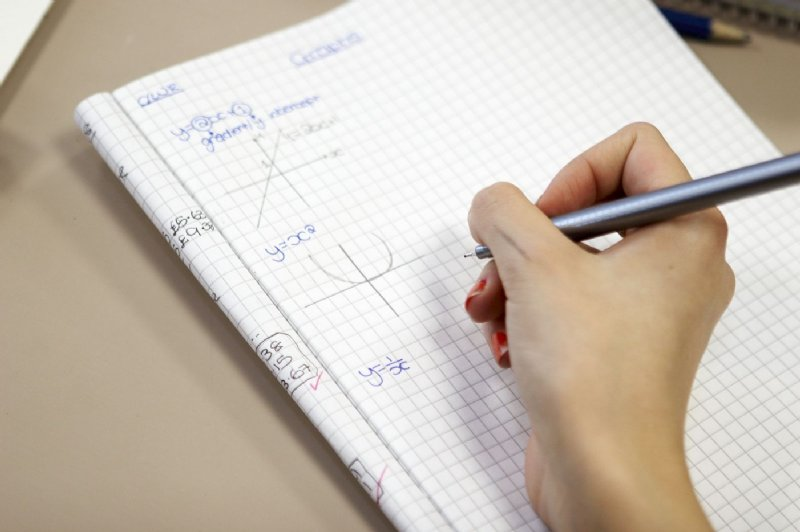High School Math 9th Grade: Evaluating and solving mathematical problems involving linear, quadratic, polynomial, exponential, logarithmic, trigonometric, and rational functions. Delineate and analyze properties of the functions through tables, graphs, or equations. 10th Grade: Geometry Measurements and relationships of segments and angles Deductive reasoning with reasoning with algebraic properties Cartesian coordinate plane to graph real-world relations11th Grade: Properties of functions Trigonometric functions and their inverses Sequences and series Polar equations Trigonometric ratios via the following formulas: sum, double angle, and half angle. Sine and cosine 12th Grade: Algebra 2: Quadratic functions and equations Graphing, and systems of linear equations and inequalities Binomial theorem Calculus: Derivatives of functions (graph, limitations or real life application) Chain rule Statistics: Finite sample spaces Probability and distribution Discrete and continuous variables and distribution Central Theorem Limit Other Topics in High School Math Trigonometry: High school trigonometry, college trigonometry, algebra trigonometry, and geometry trigonometry. Calculus: Pre-calculus, basic calculus, calculus 1, calculus 2, calculus 3, AP calculus, business calculus, multivariable calculus, advanced calculus, differential calculus, differential equations, and vector calculus.Statistics: Basic statistics, elementary statistics, high school statistics, AP. statistics, college statistics, graduate statistics, business statistics, AP. statistics, time series, and probability. Senior and Integrated Math: a combination of the above subjects including patterns and relationships, data analysis and probability and functions (linear, exponential, and quadratic).